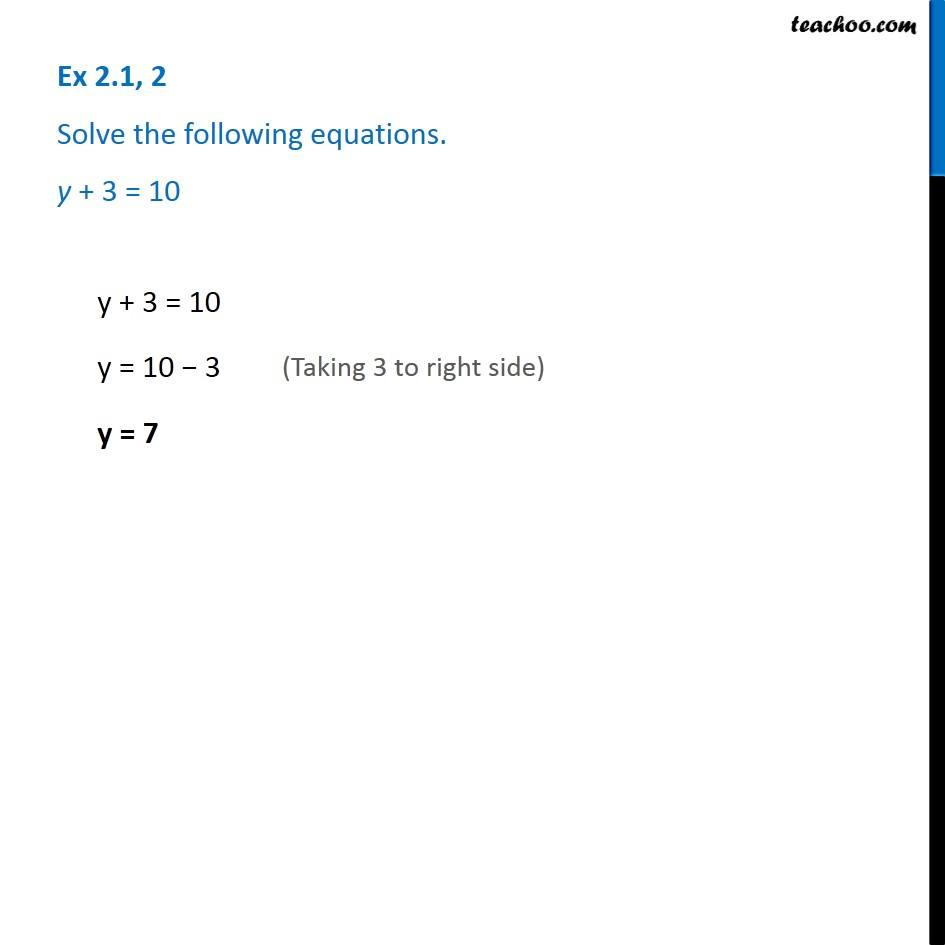Solving easy equations

Chapter 2 Class 8 Linear Equations in One Variable
Serial order wiseLearn in your speed, with individual attention - Teachoo Maths 1-on-1 Class

### Transcript

Question 2 - Chapter 2 Class 8 NCERT - Linear Equations in one variable Solve the following equations. y + 3 = 10 y + 3 = 10 y = 10 − 3 (Taking 3 to right side) y = 7